GRE Subject Test: Math : Variance

Example Questions

Example Question #1 : Variance

Find the variance of the scores 18, 4, 9, 6, 11, and 20.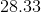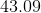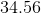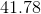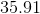Explanation:

First find the mean of the scores by adding them up and dividing by the number of scores.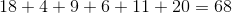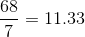Subtract the mean of 11.33 from each of the scores.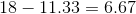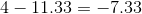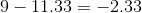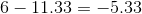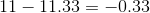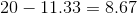Now, square the results.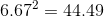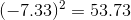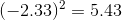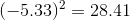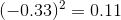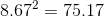Now we need the average of these values to get the variance. First, add up these values.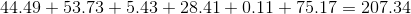Now divide by 6 which is the number of scores.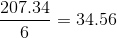Example Question #2 : Variance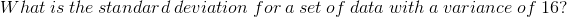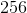Explanation: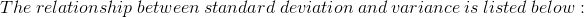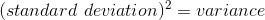or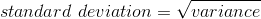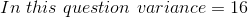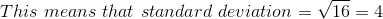All GRE Subject Test: Math Resources